#### How to Calculate Probability in Excel

You probably want to know how to calculate probability with Excel. You will after you take a look at this easy tutorial.

## PROB function in Excel

You can use the PROB function to calculate the probability in Excel. This function calculates the probability that values in a range fall within a specified range of values.

PROB function syntax:

PROB (range_x, sample_range, lower_limit, [upper_limit])

• x_range: range of x values.
• prob_range: range of x cells.
• lower_limit: the lower limit of the probability value
• upper_limit: The upper limit of the probability value is set here. This argument is optional.

The PROB function can be used to calculate the probability of values falling within any range, not just a fixed range.

## NORM.DIST Function

The NORM.DIST function calculates the cumulative probability of a random variable following a normal distribution. You can use this function to find the probability that a value falls within a specified range in a normal distribution.

Syntax: NORM.DIST(x, mean, standard_dev, cumulative)

Example: =NORM.DIST(85, 80, 10, TRUE) calculates the probability that a value less than or equal to 85 occurs in a normal distribution with a mean of 80 and a standard deviation of 10.

## NORM.INV Function

The NORM.INV function calculates the inverse of the cumulative probability for a random variable following a normal distribution. You can use this function to find the value corresponding to a specific probability in a normal distribution.

Syntax: NORM.INV(probability, mean, standard_dev)

Example: =NORM.INV(0.95, 100, 15) calculates the value corresponding to the 95th percentile in a normal distribution with a mean of 100 and a standard deviation of 15.

## Analysis Toolpak

Another method to calculate probability in Excel is to use the built-in Data Analysis Toolpak. To enable the Data Analysis Toolpak, click on “File” in the top menu, then click on “Options,” then click on “Add-Ins.” From there, select “Excel Add-ins” from the drop-down menu and click on “Go.” Check the box next to “Analysis Toolpak” and click on “OK” to enable the toolpak.

Once you have enabled the Data Analysis Toolpak, follow these steps to calculate probability:

1. Enter your data into a column in Excel.
2. Click on “Data” in the top menu and select “Data Analysis” from the drop-down menu.
3. In the “Data Analysis” dialog box, select “Descriptive Statistics” and click on “OK.”
4. In the “Descriptive Statistics” dialog box, select the range of data you want to analyze and select “Summary statistics.”
5. Check the box next to “Confidence Level for Mean” and enter the desired confidence level (usually 95%).
6. Click on “OK” to generate a summary table that includes the mean, standard deviation, and other statistical measures for your data, including the probability of a value occurring within a certain range.

Note that the Data Analysis Toolpak can also be used to perform other statistical analyses, such as regression analysis, hypothesis testing, and ANOVA.

Here are some examples of how to use the PROB function in Excel to calculate probability.

## Simple probability example

1. Enter data in Excel sheet.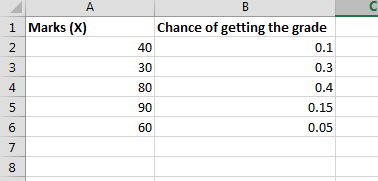2. To calculate probability, use the PROB function.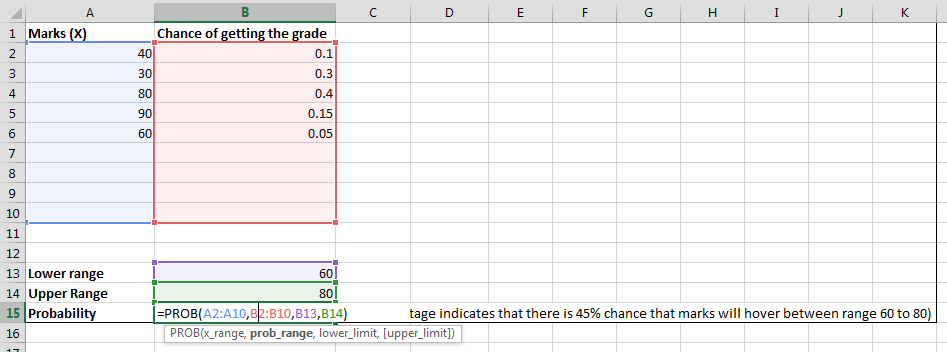The formula here is =PROB(A2:A10,B2:B10,B13,B14) where

• A2:A10 is range of marks in this example
• B2:B10 is a chance of getting the grade from A column
• B13 is lower range
• B14 stand for upper range

The probability here is 45%.

## 2 dice probability calculator

Let’s check what the probability is for rolling two dice.

I prepared data.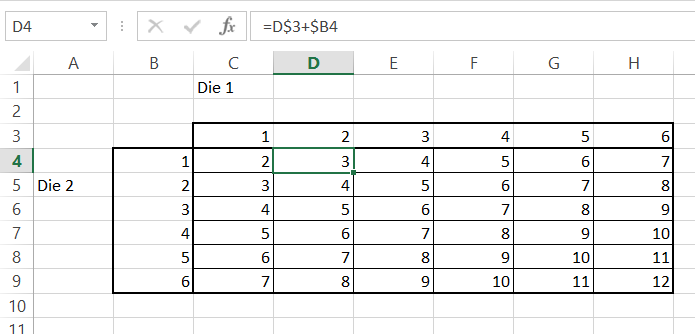First, I need to calculate the chance of rolling the die.

It is possible to roll from 2 to 12 with two dice. The roll chance formula is: =COUNTIF(\$C\$4:\$H\$9,K4)

I used absolute reference in the formula.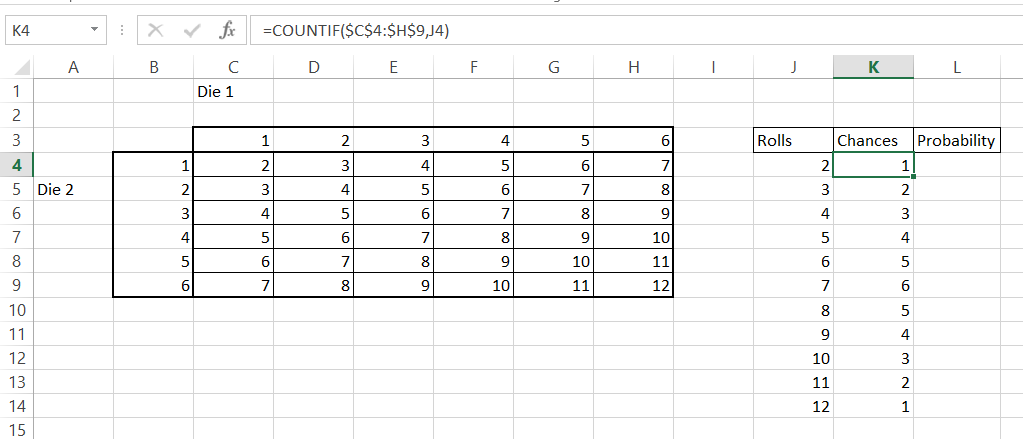There are:

• only one chance to roll 2 and 12
• two chances to roll 3 and 11
• three chances to roll 4 and 10
• four chances to roll 5 and 9
• five chances to roll 6 and 8
• six chances to roll 7

To calculate the probability of 2 dice, you need to divide the number of chances by the sum of chances.

The probability formula for two dice is=\$K4/SUM(\$K\$4:\$K\$14)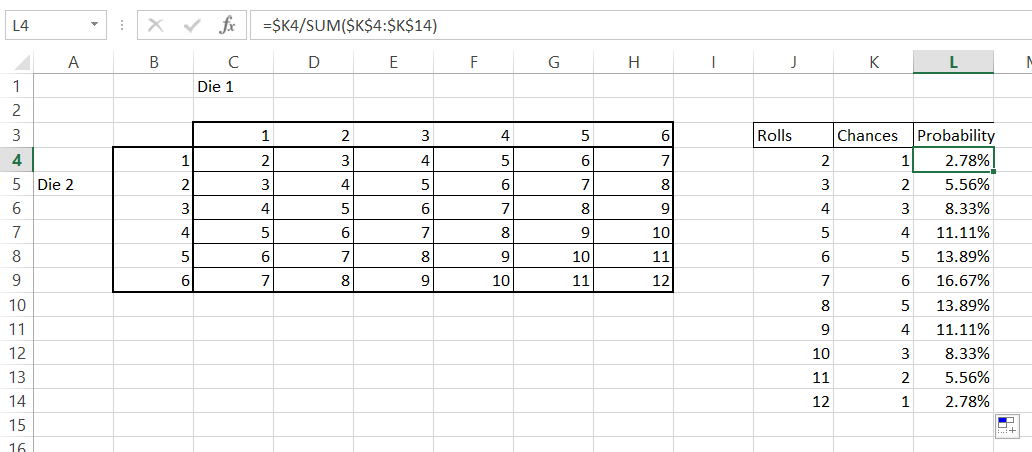Two dice probabilities are from 2.78% to 16.67%.

Based on the above calculations, you can easily calculate what the probability of rolling doubles is.

There are only six chances to do that, so the probability of rolling doubles is 6/36 = 16.67%. The interesting thing is that the probability of rolling doubles is exactly the same as rolling 7.

## The probability of a coin flip

It is 50% to flip the head and 50% to flip the tail. Have you ever wondered what the probability is of flipping the head (or tail) 11 times in a row? Let’s calculate it in Excel.

First, let’s calculate the probability of flipping a head once. It is easy. 50%. head or flip.

By translating it to the Excel language, we can create an Excel formula: =POWER(0.5,1)

Further, the probability is equal to =POWER(0.5, number_of_tries).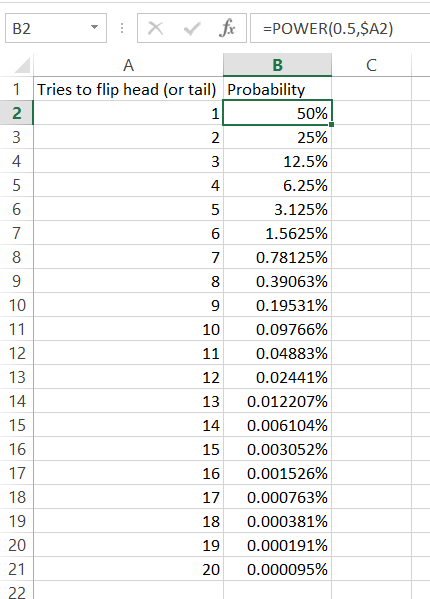The probability of flipping a head (or tail) 11 times in a row equals 0.04883%, which is less than 0.05%!

Flipping a head 10 times is 0.09766% probable.

Flipping your head (or tail) 20 times in a row is 0.000095% probable.

We can also look for the possibility of flipping heads (or tails) several times in a row. It’s easy because each time is 2 times less probable.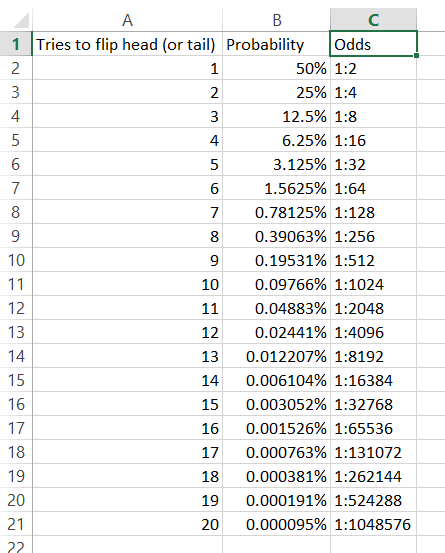It’s 1: 2048 odds of flipping a head (or tail) 11 times in a row. It means that every 2048 times you flip a coin, 11 times you flip 11 heads (or tails).

## Poker probability

It will be fun to analyze the poker probabilities.

First, use the Excel function to check the number of poker combinations. There are 52 cards, from which you get only 5. From a mathematical point of view, it is a simple combination to be used. There is an Excel function COMBIN, for that. Functions take just two arguments as a combination. Simply use the Excel formula =COMBIN(52,5)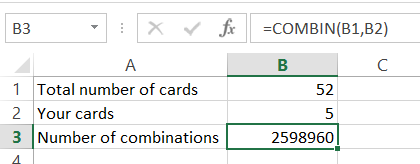It turns out there are 2,598,960 combinations in poker.

To calculate the probability of getting a pair or two pairs, you need to calculate the number of combinations of getting them. And then just divide the two numbers.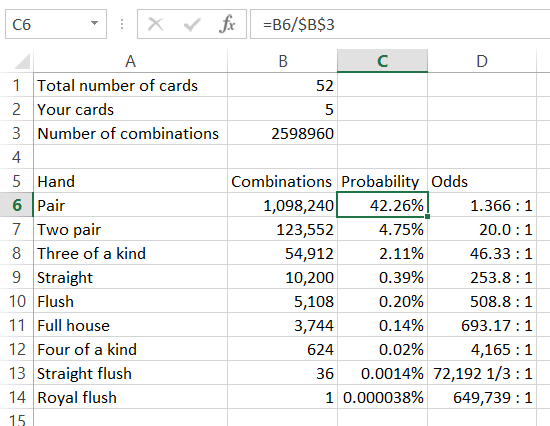The probability is:

• To get a pair 42.26%
• To get two pair 4.75
• For a three of a kind 2.11%
• Straight 0.39%
• Full house 0.2%
• Four of a kind 0.14%
• Four of a kind 0.02%
• Straight flush 0.0014%
• Royal flush 0.000038% (1:649,736 odds)